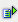The function exp (num) potentialized the basis e with the number stated as argument.

Syntax: =exp(num)Example =exp(0) is 1 =exp(1) is 2.718282 =exp(10) is 22026,465795